In: Chemistry

# For 1 mol of an ideal gas, Pexternal = P = 1 atm. The temperature is...

For 1 mol of an ideal gas, Pexternal = P = 1 atm. The temperature is changed from 125ºC to 25.0ºC, and CV,m = 3/2R. Calculate (all units are J) q= , w= , ∆U= , and ∆H= . Please enter your answers with 2 decimals in E notation, such as 2.33E4 (=23345). If the answer is negative, please do not forget the negative sign. If answer is zero, please just enter 0 without decimal.

## Solutions

##### Expert Solution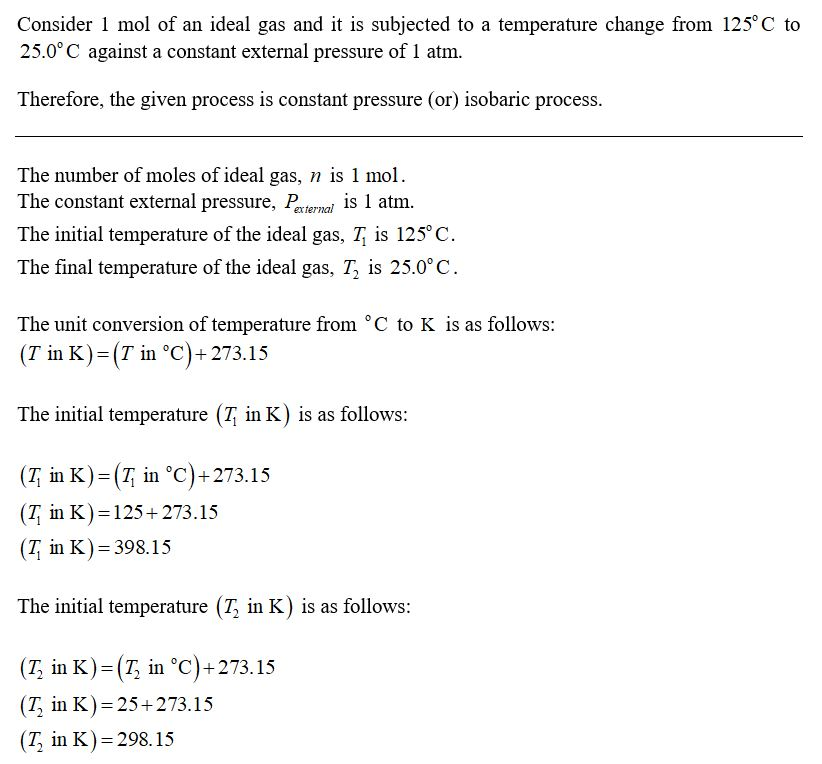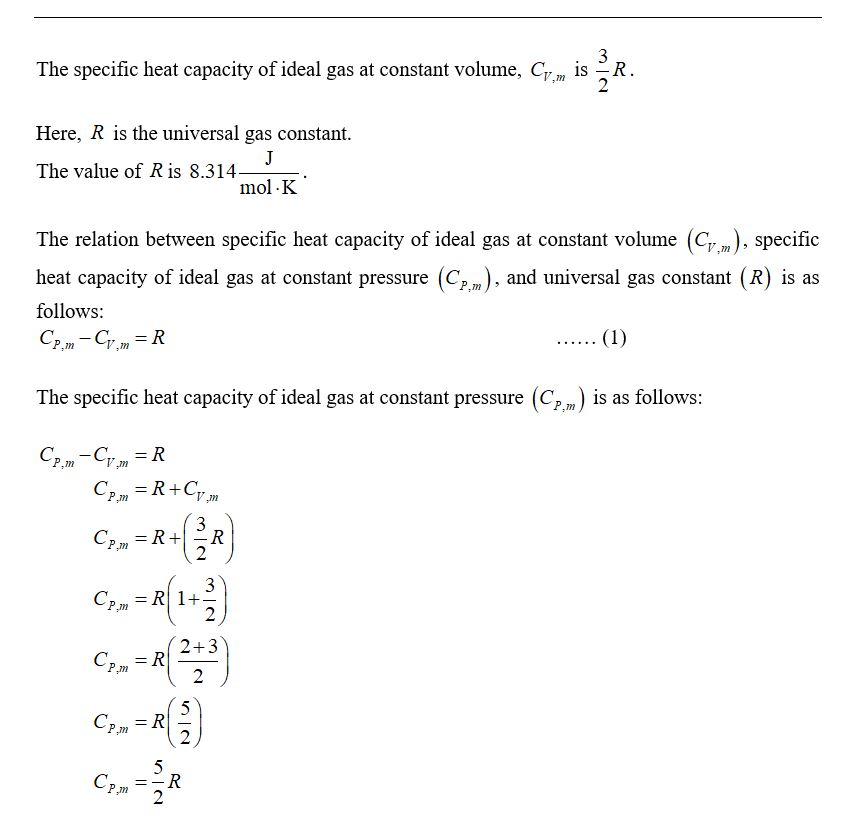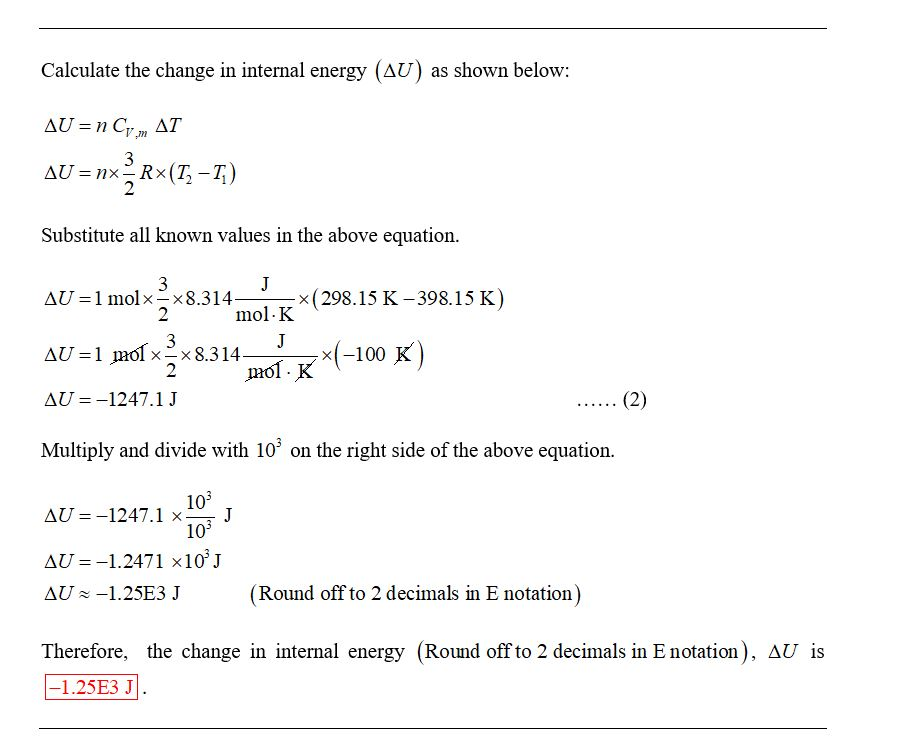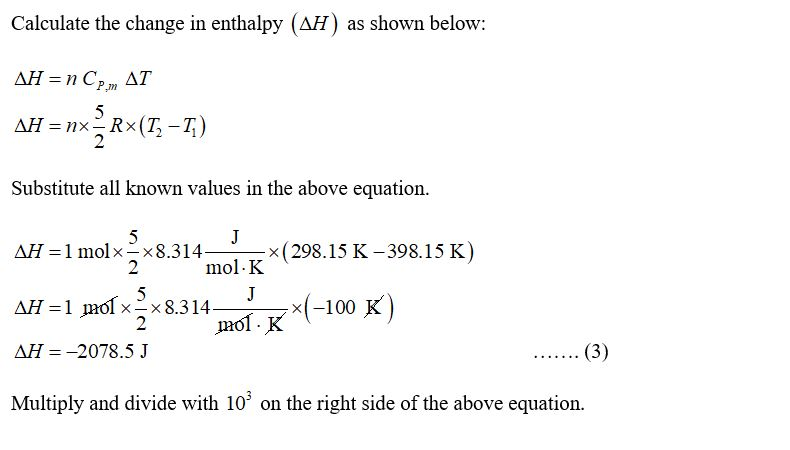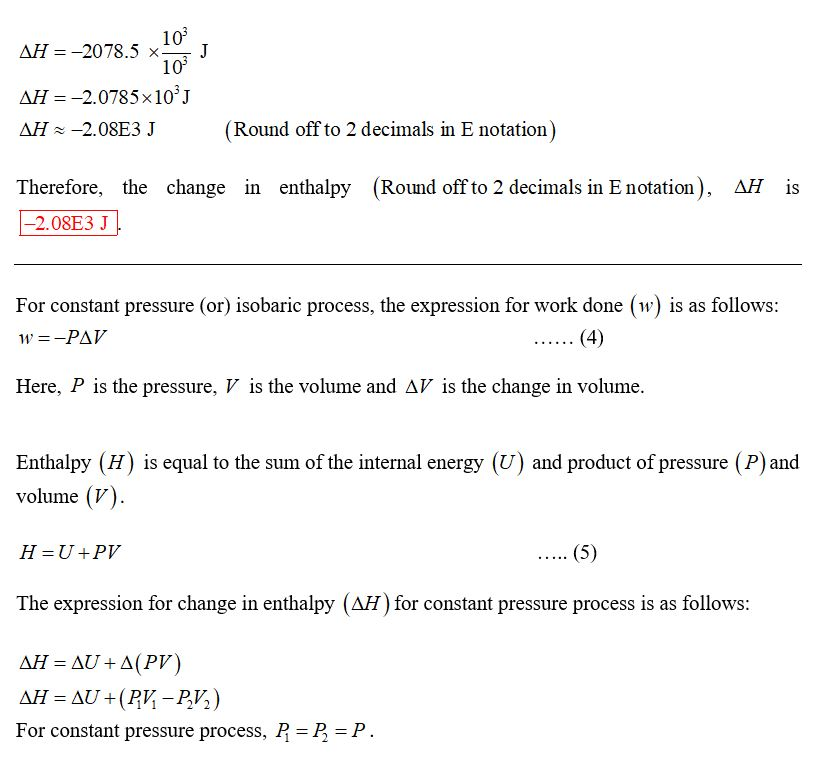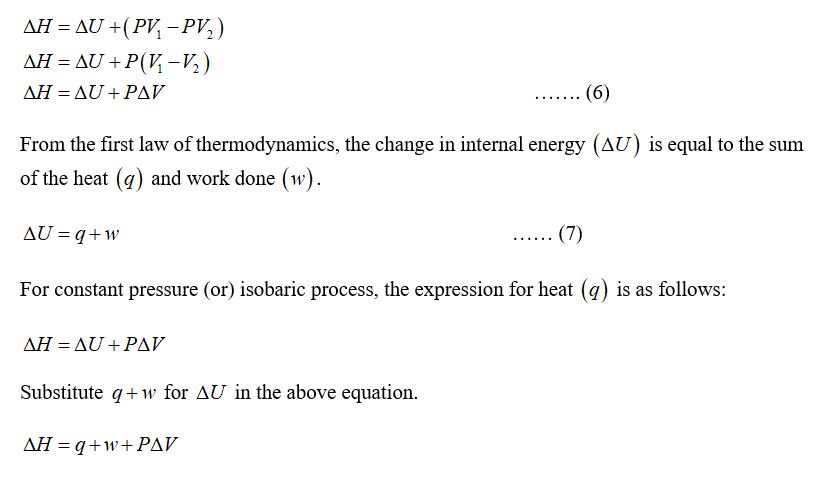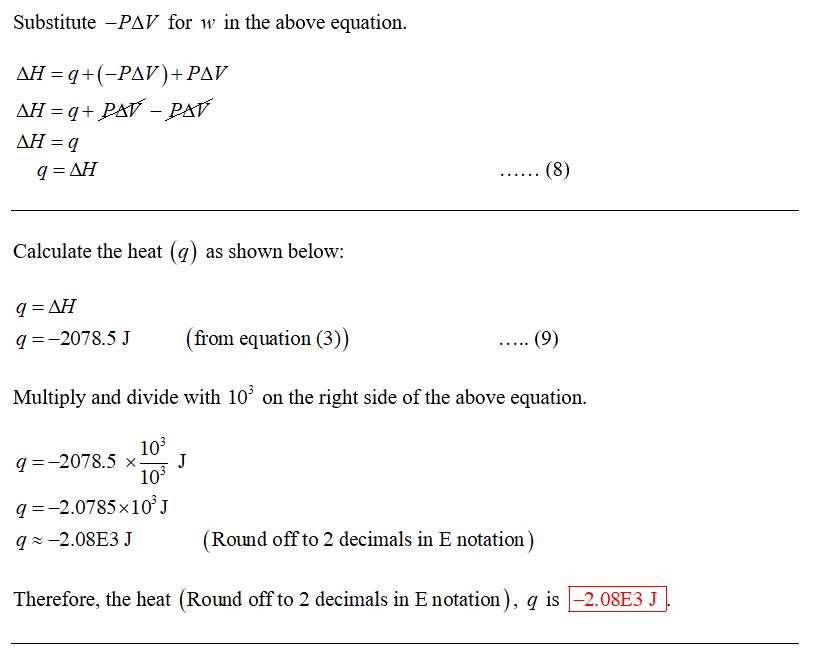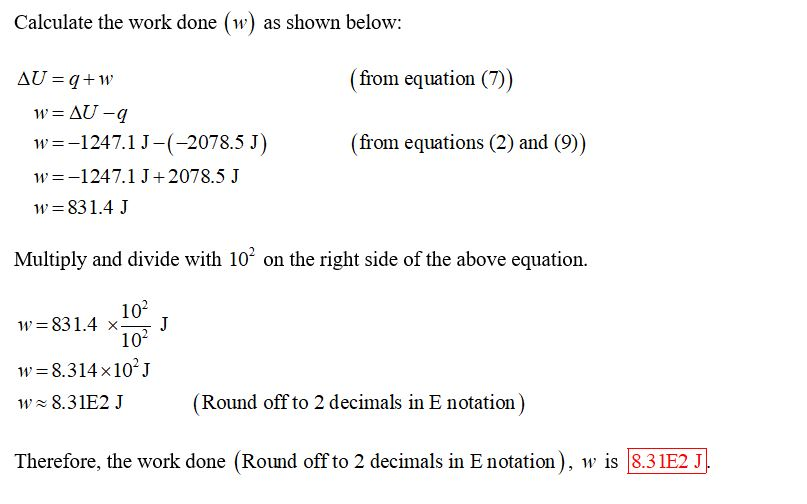## Related Solutions

##### Suppose 1.0 mol of an ideal gas is initially at P=4.0 atm and T=400 K. It...
Suppose 1.0 mol of an ideal gas is initially at P=4.0 atm and T=400 K. It is expanded irreversibly and adiabatically against a constant pressure of 1.0 atm until the volume has doubled. (a) Calculate the final volume of the gas. (b) Calculate w, q, and energy change ΔU of this process, in joules. (c) Calculate the final temperature of the gas. (d) Calculate the entropy change ΔS of the ideal gas in the process. (e) What is the entropy...
##### The temperature of 2.00 mol of an ideal monatomic gas is raised 15.0 K at constant...
The temperature of 2.00 mol of an ideal monatomic gas is raised 15.0 K at constant volume. What are (a) the work W done by the gas, (b) the energy transferred as heat Q , (c) the change ?Eint in the internal energy of the gas, and (d) the change ?K in the average kinetic energy per atom
##### The pressure on a sample of an ideal gas is increased from 715 mmHg to 3.55 atm at constant temperature.
The pressure on a sample of an ideal gas is increased from 715 mmHg to 3.55 atm at constant temperature. If the initial volume of the as is 472 mL. what is the final volume of the gas?   A 4.00 L sample of gas is cooled from 71 CC to a temperature at which its volume is 2.60 L. What is this new temperature? Assume no change in pressure of the gas.   A scuba diver releases a...
##### 3 moles of an ideal gas with 2 atoms under 308 K temperature and 5 atm...
3 moles of an ideal gas with 2 atoms under 308 K temperature and 5 atm are expanded reversible to 1 atm end pressure. Calculate w, q, ΔU, ΔH values ​​in case of expansion of this expansion by isothermal and adiabatic way.
##### 1. A sample of nitrogen gas at a pressure of 0.947 atm and a temperature of...
1. A sample of nitrogen gas at a pressure of 0.947 atm and a temperature of 213 °C, occupies a volume of 687 mL. If the gas is cooled at constant pressure until its volume is 570 mL, the temperature of the gas sample will be 2. A helium-filled weather balloon has a volume of 615 L at 23 °C and 754 mm Hg. It is released and rises to an altitude of 7.93 km, where the pressure is 323...
##### Use the ideal gas law to complete the table: P V n T 1.06 atm 1.25...
Use the ideal gas law to complete the table: P V n T 1.06 atm 1.25 L 0.105 mol ___ 115 torr ___ 0.249 mol 309 K ___ 28.2 mL 1.81×10−3 mol 26.0 ∘C 0.565 atm 0.440 L ___ 257 K
##### A sample of an ideal gas at 1.00 atm and a volume of 1.46 L was...
A sample of an ideal gas at 1.00 atm and a volume of 1.46 L was placed in a weighted balloon and dropped into the ocean. As the sample descended, the water pressure compressed the balloon and reduced its volume. When the pressure had increased to 10.0 atm, what was the volume of the sample? Assume that the temperature was held constant.
##### A sample of an ideal gas at 1.00 atm and a volume of 1.02 L was...
A sample of an ideal gas at 1.00 atm and a volume of 1.02 L was placed in a weighted balloon and dropped into the ocean. As the sample descended, the water pressure compressed the balloon and reduced its volume. When the pressure had increased to 45.0 atm, what was the volume of the sample? Assume that the temperature was held constant.
##### Gas is confined in a tank at a pressure of 11.5 atm and a temperature of...
Gas is confined in a tank at a pressure of 11.5 atm and a temperature of 22.0°C. If two-thirds of the gas is withdrawn and the temperature is raised to 60.0°C, what is the pressure of the gas remaining in the tank?
##### 15 mol /h of stream 1 (gas, at 1.1 atm and 0°C) containing 25% (mol basis)...
15 mol /h of stream 1 (gas, at 1.1 atm and 0°C) containing 25% (mol basis) propane and rest butane is mixed with a second stream (gas, at 1.1 atm and 70°C) containing 40% (mol basis) propane and rest butane. The output stream is 40 mol/h (gas, at 1.1 atm). The relative enthalpies as a function of temperature (T is in °C) are H (J/mol) for propane= 91*T H (J/mol) for butane= 121*T a) What is the composition of the...